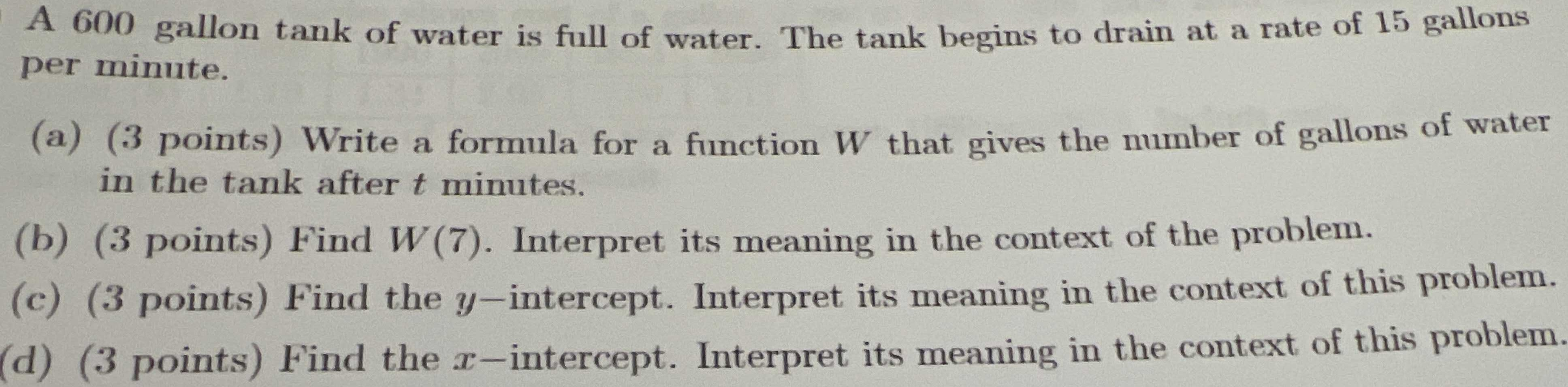### ¿Todavía tienes preguntas de matemáticas?

Pregunte a nuestros tutores expertos
Algebra
Pregunta$$600$$ gallon tank of water is full of water. The tank begins to drain at a rate of $$15$$ gallons per minute.

(a) ( $$3$$ points) Write a formula for a function $$W$$ that gives the number of gallons of water in the tank after $$t$$ minutes.

(b) ( $$3$$ points) Find $$W ( 7 )$$ . Interpret its meaning in the context of the problem.

(c) ( $$3$$ points) Find the $$y$$ -intercept. Interpret its meaning in the context of this problem.

(d) (3 points) Find the $$x$$ -intercept. Interpret its meaning in the context of this problem